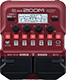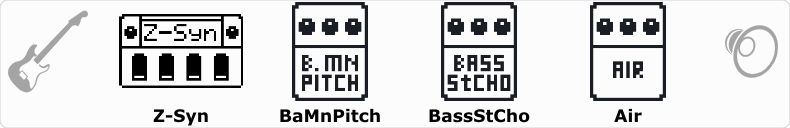# ZBass0

Discussion in 'Zoom B1/B1X Four' started by prea, Jan 25, 2022.

1. ZBass0Device: Zoom B1 Four
Firmware: 2.00

Name on device: Z-Synth
Optimized for: Phones/Speaker

Effects chain:Though Z-synth test patch ...

Effect: "Z-Syn" (Sfx), active - "yes"
"FREQ" = 4
"Range" = 13
"Decay" = 0
"RESO" = 0
"Wave" = 1
"Tone" = 1
"BAL" = 95
"VOL" = 10
"Frequency" = 0
"Range" = 0
"Decay" = 0
"Resonance" = 0
"Wave" = SAW
"Tone" = 0
"Balance" = 0
"Volume" = 0

Effect: "BaMnPitch" (Modulation), active - "yes"
"Shift" = 12
"Fine" = 0
"Tone" = 2
"Balance" = 22

Effect: "BassStCho" (Modulation), active - "yes"
"Depth" = 75
"Rate" = 27
"LoCut" = 180 Hz
"Mix" = 76

Effect: "Air" (Reverb), active - "yes"
"Size" = 10
"REF" = 0
"Mix" = 60
"Tail" = On

Patch Volume: 87